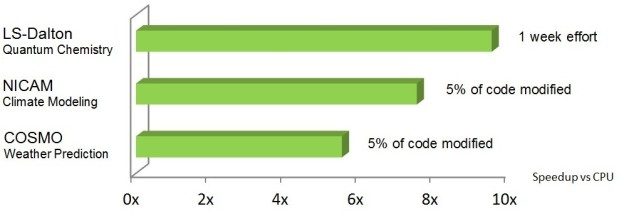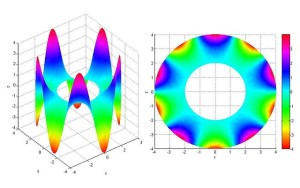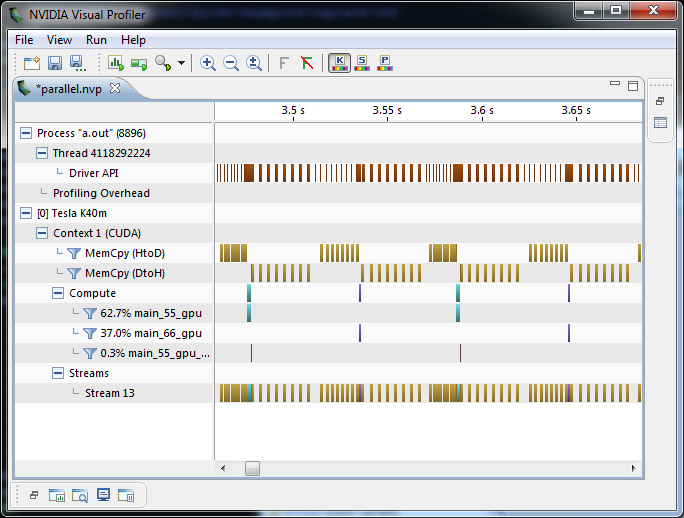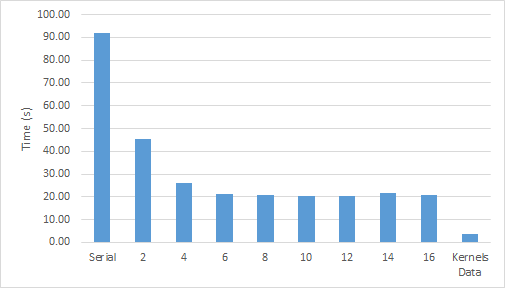# Getting Started with OpenACCThis week NVIDIA has released the NVIDIA OpenACC Toolkit, a starting point for anyone interested in using OpenACC. OpenACC gives scientists and researchers a simple and powerful way to accelerate scientific computing without significant programming effort. The toolkit includes the PGI OpenACC Compiler, the NVIDIA Visual Profiler with CPU and GPU profiling, and the new OpenACC Programming and Best Practices Guide. Academics can get a free renewable license to the PGI C,C++ and Fortran compilers with support for OpenACC.Figure 1: LS-DALTON: Benchmark on Oak Ridge Titan Supercomputer, AMD CPU vs Tesla K20X GPU. Test input: Alanine-3 on CCSD(T) module. Additional information: NICAM COSMO
OpenACC tutorial: Three Steps to More Science

OpenACC is an open specification for compiler directives for parallel programming. By using OpenACC, developers can rapidly accelerate existing C, C++, and Fortran applications using high-level directives that help retain application portability across processor architectures. Figure 1 shows some examples of real code speedups with OpenACC. The OpenACC specification is designed and maintained with the cooperation of many industry and academic partners, such as Cray, AMD, PathScale, University of Houston, Oak Ridge National Laboratory and NVIDIA.

When I program with and teach OpenACC I like to use a 4 step cycle to progressively accelerate the code.

1. Identify Parallelism: Profile the code to understand where the program is spending its time and how much parallelism is available to be accelerated in those important routines. GPUs excel when there’s a significant amount of parallelism to exploit, so look for loops and loop nests with a lot of independent iterations.
2. Express Parallelism: Placing OpenACC directives on the loops identified in step 1 tells the compiler to parallelize them. OpenACC is all about giving the compiler enough information to effectively accelerate the code, so during this step I add directives to as many loops as I believe I can and move as much of the computation to the GPU as possible.
3. Express Data Locality: The compiler needs to know not just what code to parallelize, but also which data will be needed on the accelerator by that code. After expressing available parallelism, I often find that the code has slowed down. As you’ll see later in this post, this slowdown comes from the compiler making cautious decisions about when data needs to be moved to the GPU for computation. During this step, I’ll express to the compiler my knowledge of when and how the data is really needed on the GPU.
4. Optimize – The compiler usually does a very good job accelerating code, but sometimes you can get more performance by giving the compiler a little more information about the loops or by restructuring the code to increase parallelism or improve data access patterns. Most of the time this leads to small improvements, but sometimes gains can be bigger.

It’s really important after each of these steps to verify that the program still produces correct results, as it’s much easier to debug a mistake due to one small change to the code rather than waiting to the end after making many changes. This process is a cycle, so once you have an important hotspot running well with OpenACC, go back to the beginning and find the next place to express parallelism.

In this post I’ll review steps 2, 3, and 4 using a simple benchmark code, but first, let’s install the NVIDIA OpenACC Toolkit. If you already have an OpenACC compiler installed on your machine, you can skip this step.

## Installing the OpenACC Toolkit

You can download the OpenACC Toolkit from https://developer.nvidia.com/openacc by clicking the download link. In order to install it on your machine, you will need to be running a 64-bit Linux OS and have a CUDA-capable GPU installed. Once you’ve downloaded the package, untar it into a temporary directory and run the `install` script. When asked whether to install the CUDA Toolkit Components, be sure to answer yes, as they are required by the OpenACC compiler. If you’ve never installed the CUDA Toolkit on your machine, you will need to take one additional step: installing the NVIDIA CUDA driver. This driver is included in the installation directory, which defaults to `/opt/pgi`. Within that directory, go to the `linux86-64/2015/OpenACC/driver` directory and use `bash ./*.run` to install the CUDA driver or head to https://developer.nvidia.com/cuda-downloads to install the CUDA Toolkit and driver via your Linux package manager. This will walk you through installing the driver. If you have any trouble with this step, please post a question on the NVIDIA forums.

Before moving forward, be sure to take a look at the documentation included in the Toolkit installation directory under the `linux86-64/2015/OpenACC/doc` directory.

## Jacobi IterationFigure 2: Laplace’s Equation on a ring with boundary conditions. Laplace Equation Image via WikiMedia Commons

The benchmark I will use is a simple C code that solves the 2D Laplace equation with the iterative Jacobi solver. Iterative methods are a common technique to approximate the solution of elliptic PDEs, like the Laplace equation, within an allowable tolerance. In the example I’ll perform a simple stencil calculation where each point calculates its value as the mean of its neighbors’ values. The Jacobi iteration continues until either the maximum change in value between two iterations drops below the tolerance level or it exceeds the maximum number of iterations. For the sake of consistent comparison all the results I show are for 1000 complete iterations. Here’s the main Jacobi iteration loop.

```while ( error > tol && iter < iter_max ) {
error = 0.0;
for( int j = 1; j < n-1; j++) {
for( int i = 1; i < m-1; i++ ) {
Anew[j][i] = 0.25 * ( A[j][i+1] + A[j][i-1] +
A[j-1][i] + A[j+1][i]);
error = fmax( error, fabs(Anew[j][i] - A[j][i]));
}
}

for( int j = 1; j < n-1; j++) {
for( int i = 1; i < m-1; i++ ) {
A[j][i] = Anew[j][i];
}
}

if(iter % 100 == 0) printf("%5d, %0.6f\n", iter, error);
iter++;
}
```

The outer loop iterates for 1000 iterations or until the results converge to within an acceptable tolerance (whichever comes first). I’ll refer to this loop as the convergence loop. The two `j`, `i` loop nests within the convergence loop do the main calculation. The first loop calculates the new values for each point in the matrix as the mean of the neighboring values. The second loop nest copies these values into the `A` array for the next iteration. Note that the code calculates an `error` value for each element, which is how much the value changed between iterations, and finds the maximum value of `error` to determine whether the solution has converged.

## Express Parallelism

In this code it’s clear that the convergence loop cannot be parallelized because of the dependence of each iteration on the results of the previous. This is known as a data dependency. The two inner loop nests over `i` and `j`, however, can be parallelized, as each iteration writes its own unique value and does not read from the values calculated in other iterations. These loops can be executed forward, backward, in a random order, or all simultaneously and the results will be the same (modulo floating point error). Therefore, the two loop nests are candidates for acceleration. I’ll use the OpenACC `kernels` directive to accelerate them. The `kernels` directive tells the compiler to analyze the code in the specified region to look for parallel loops. In the following code, I’ve placed a `kernels `region within the convergence loop, around the two loop nests that perform the calculation and value swapping, since this is where the compiler is most likely to find parallelism.

```while ( error > tol && iter < iter_max ) {
error = 0.0;
#pragma acc kernels
{
for( int j = 1; j < n-1; j++) {
for( int i = 1; i < m-1; i++ ) {
Anew[j][i] = 0.25 * ( A[j][i+1] + A[j][i-1]
+ A[j-1][i] + A[j+1][i]);
error = fmax( error, fabs(A[j][i] - Anew[j][i]));
}
}

for( int j = 1; j < n-1; j++) {
for( int i = 1; i < m-1; i++ ) {
A[j][i] = Anew[j][i];
}
}
}

if(iter % 100 == 0) printf("%5d, %0.6f\n", iter, error);
iter++;
}
```

Having inserted an OpenACC directive I now want to build the code. For this I’ll use the PGI C/C++ compiler, `pgcc`, which is included in the OpenACC Toolkit. I want the compiler to generate code specific to NVIDIA GPUs, so I add the `-ta=tesla` compiler option (`ta` means target accelerator and `tesla` targets NVIDIA Tesla GPUs). I also use the optional `-Minfo=accel` compiler flag to tell the compiler to provide feedback about the code it generates. Below is the output from this command.

```\$ pgcc -acc -ta=tesla -Minfo=accel laplace2d-kernels.c
main:
56, Generating copyout(Anew[1:4094][1:4094])
Generating copyin(A[:][:])
Generating copyout(A[1:4094][1:4094])
Generating Tesla code
58, Loop is parallelizable
60, Loop is parallelizable
Accelerator kernel generated
58, #pragma acc loop gang /* blockIdx.y */
60, #pragma acc loop gang, vector(128) /* blockIdx.x threadIdx.x */
64, Max reduction generated for error
68, Loop is parallelizable
70, Loop is parallelizable
Accelerator kernel generated
68, #pragma acc loop gang /* blockIdx.y */
70, #pragma acc loop gang, vector(128) /* blockIdx.x threadIdx.x */
```

Notice from the compiler output that the compiler identified two regions of code for generating an accelerator kernel. (A kernel is simply a function that is run in parallel on the accelerator / GPU). In this case, the loop nests starting at lines 58 and 68 have been identified as safe to parallelize and the compiler has generated kernels for these loops. The compiler also analyzed which arrays are used in the calculation and generated code to move `A` and `Anew` into GPU memory. The compiler even detected that it needs to perform a max reduction on the `error` variable.

A reduction is an operation that takes many input values and combines them into a single result using a binary operator. In this c+ase, each loop iteration calculates an `error `value, but the iteration logic only needs one result: the maximum error. Reductions can be tricky in some parallel programming languages, but fortunately the OpenACC compiler handled the reduction for me. Now that I’ve built the code, I can expect it to run significantly faster on my GPU, right? Figure 3 shows the performance of this code running on multiple cores of an Intel Xeon E5-2698 CPU to performance on an NVIDIA Tesla K40 GPU. The CPU code was parallelized using OpenMP and compiled with PGI 15.5 and `-fast` optimizations.Figure 3: OpenACC Jacobi Iteration after adding a kernels directive (rightmost bar), compared to the original serial code on the CPU and accelerated with OpenMP (2-16 CPU threads).

Figure 1 clearly shows a speedup from increasing the number of CPU threads—although not for more than 8 threads—but what about the GPU performance? The OpenACC code on the GPU slightly outperforms the original, single-threaded version, but not the multi-threaded version. I used the NVIDIA Visual Profiler to help understand what’s happening. Figure 4 shows the GPU timeline, zoomed in to just 2 iterations of the convergence loop. The profiler shows the kernels of the two loop nests in green and purple, but the run time of the kernels is overshadowed by the tan boxes that represent PCIe data copies (host to device and device to host). Now that I know the problem, I can fix it.Figure 4: Running the NVIDIA Visual Profiler on the  OpenACC Jacobi iteration code after adding a kernels directive shows that run time is dominated by PCI-express data transfers.

## Express Data Locality

Why is PCIe data transfer time overwhelming the speed-up from GPU execution of the Jacobi iteration? Looking closely at the Visual Profiler timeline it appears that the code copies data to the GPU each time it enters the `kernels` region, and copies the data back to the CPU each time it exits the `kernels` region. Looking at the algorithm, however, I only really need the initial value of `A` copied to the device at the beginning of the convergence loop and the final value copied out at the end. The data doesn’t change between iterations of the `while` loop, so I can leave the arrays on the device. As the programmer, it’s easy for me to see this because I know the algorithm, but it’s very difficult for the compiler. Compilers can’t afford to produce wrong answers, so they’d rather copy the data too often than not copy it and produce incorrect results. In fact, the OpenACC specification requires the compiler to default to copying arrays to and from the device at every `kernels` region when the programmer doesn’t specify how to handle them. Fortunately, OpenACC provides a way to tell the compiler when data really needs to move. The OpenACC `data` construct specifies data movement or residency options for a region of code, using clauses that inform the compiler explicitly how to handle arrays, as shown in the following code.

```#pragma acc data copy(A) create(Anew)
while ( error > tol && iter < iter_max )
{
error = 0.0;

#pragma acc kernels
{
for( int j = 1; j < n-1; j++) {
for( int i = 1; i < m-1; i++ ) {
Anew[j][i] = 0.25 * ( A[j][i+1] + A[j][i-1]
+ A[j-1][i] + A[j+1][i]);
error = fmax( error, fabs(A[j][i] - Anew[j][i]));
}
}

for( int j = 1; j < n-1; j++) {
for( int i = 1; i < m-1; i++ ) {
A[j][i] = Anew[j][i];
}
}
}

if(iter % 100 == 0) printf("%5d, %0.6f\n", iter, error);
iter++;
}
```

I added a `data` region around the `while` loop, since this is where I can reuse the data on the GPU. I used the `copy` clause to tell the compiler that it should copy the A array to and from the device as it enters and exits the region, respectively. Since the `Anew` array is only used within the convergence loop, I tell the compiler to `create` temporary space on the device, since I don’t care about the initial or final values of that array. There are other `data` clauses that I don’t use in this example:

• `copyin` initializes the value of the array but does not copy the results back;
• `copyout` allocates uninitialized space on the device and copies the final values back; and
• `present` tells the compiler to assume that the array was moved or allocated on the device somewhere else in the program.

This single-line data clause makes a big difference to performance. Figure 5 shows that with data locality properly expressed the code runs six times faster than a full CPU socket with almost no data motion.Figure 5: OpenACC Jacobi Iteration after adding a `data` region to optimize data locality (rightmost bar), compared to the original serial code on the CPU and accelerated with OpenMP (2-16 CPU threads).

Figure 6 shows the NVIDIA Visual Profiler timeline, which shows that there’s no data motion between convergence iterations, just as expected.

The third step in the OpenACC APOD process is to further optimize the loops, but it turns out that the PGI compiler already does a great  job optimizing this code and there’s not much more that we can do. If you’d like to see more about optimizing your OpenACC code, however, you should check out my Advanced OpenACC Programming session from GTC.

## Optimize

Given the simplicity of this code, the compiler already does a pretty good job generating fast code, but there’s still a little room for optimization. After some experimentation I found that by using the `loop tile` clause I can reduce the runtime by a few percent. The `loop` directive is a way to give the compiler additional information about the following loop, such as informing the compiler that all loop iterations are data independent, or guiding the compiler’s optimization within the loop.

It turns out that there’s a lot of data reuse between loop iterations in the `i` and `j` dimensions of `A`, so we’ll use the tile directive to tell the compiler it should operate on tiles of the array, rather than parallelizing strictly over columns. If I wanted to do this manually, I’d have to add two more loops to the calculation to break the work up into chunks (as one might when optimizing for CPU cache blocking), but the `tile` clause tells the compiler to do it automatically. On my machine, a 32×4 tile gives the best performance, reducing the runtime by an additional 6%. Below is the resulting code. Notice that I added a `device_type(nvidia)` clause to my `loop` directives, which informs the compiler to only apply this optimization on NVIDIA GPUs, where I’ve confirmed that it’s beneficial. Using the `device_type` clause helps keep my code portable to other devices.

```#pragma acc data copy(A) create(Anew)
while ( error > tol && iter < iter_max )
{
error = 0.0;

#pragma acc kernels
{
#pragma acc loop tile(32,4) device_type(nvidia)
for( int j = 1; j < n-1; j++) {
for( int i = 1; i < m-1; i++ ) {
Anew[j][i] = 0.25 * ( A[j][i+1] + A[j][i-1]
+ A[j-1][i] + A[j+1][i]);
error = fmax( error, fabs(A[j][i] - Anew[j][i]));
}
}

#pragma acc loop tile(32,4) device_type(nvidia)
for( int j = 1; j < n-1; j++) {
for( int i = 1; i < m-1; i++ ) {
A[j][i] = Anew[j][i];
}
}
}

if(iter % 100 == 0) printf("%5d, %0.6f\n", iter, error);
iter++;
}
```

## Try OpenACC Today!OpenACC is a powerful, portable way to accelerate applications with GPUs. Get started by downloading the OpenACC Toolkit. While it’s downloading, sign up for the upcoming webinar Getting Started with PGI OpenACC Compiler, presented by PGI’s Michael Wolfe, and put it on your calendar for August 11 at 9AM Pacific.

Learn OpenACC hands-on with a self-paced lab that runs entirely in your browser (no GPU required). You can learn even more by watching OpenACC content on GTC on Demand, and by reading past Parallel Forall blog posts: